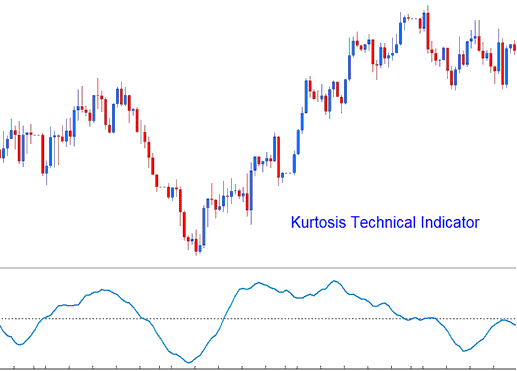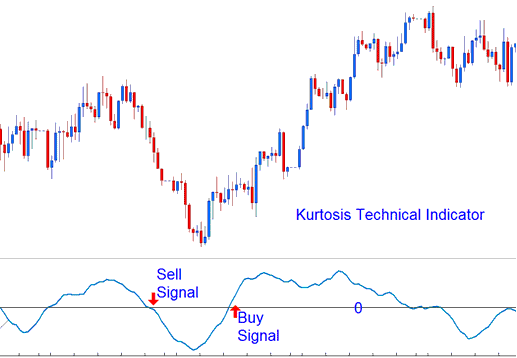This indicator is used to help determine the sentiment of the xauusd market.

It is calculated in 3 parts.

• Kurtosis portion

• Fast Kurtosis

• Fast/Slow Kurtosis

The Kurtosis portion is calculated using the formula:

K = n-periods momentum - n-periods momentum of 1 bar before (current xauusd price bar’s momentum value minus the previous xauusd price bar’s momentum value)

The Fast Kurtosis portion is calculated using the formula:

FK = n-periods moving average of K

The Fast/Slow Kurtosis portion is calculated using the formula:

FSK = n-periods moving average of FKBullish Signal - A buy gold signal is generated when the indicator crosses above zero.

Bearish Signal - A sell gold signal is generated when the indicator crosses below zero.Technical Analysis